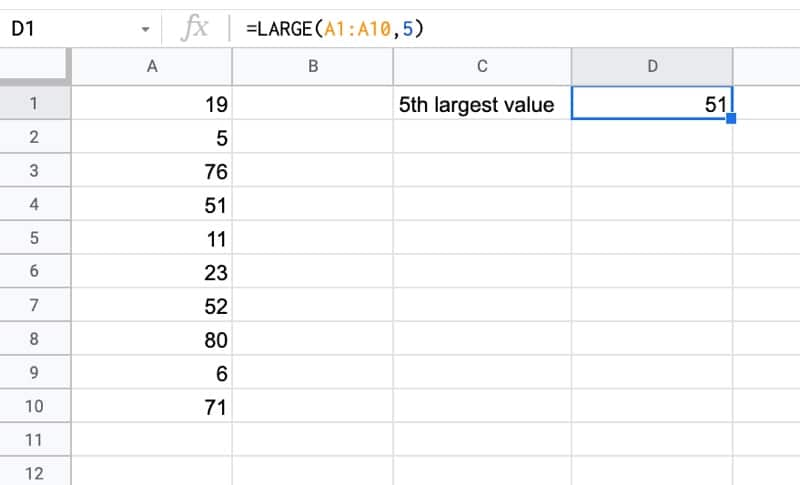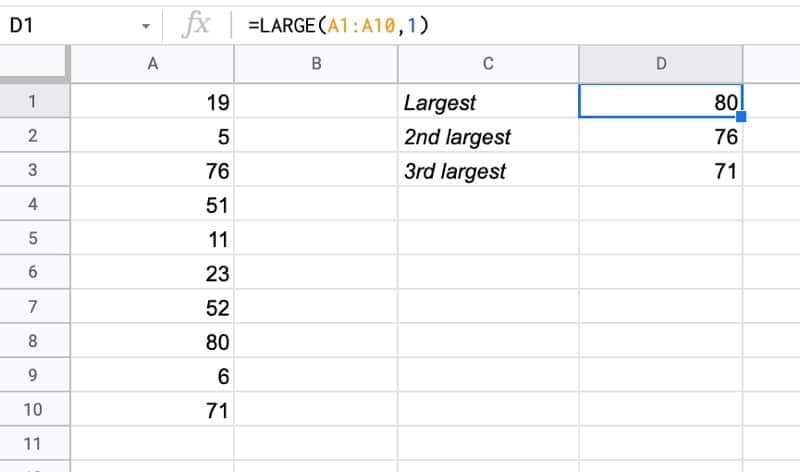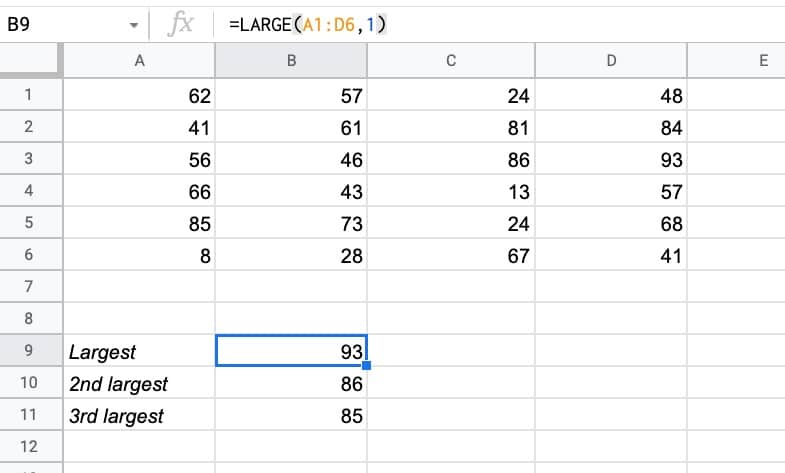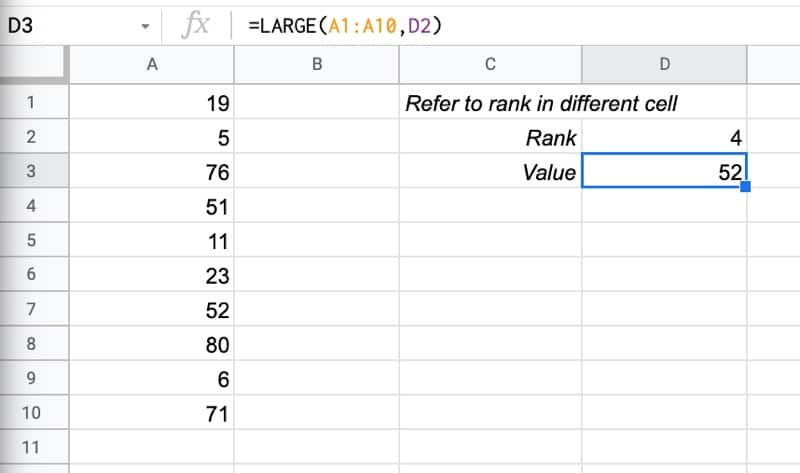# The LARGE Function In Google Sheets

The LARGE function in Google Sheets returns the n-th largest value from a dataset.

For example, you could use large to determine the 5th largest value in a dataset, the 10th largest, the 50th largest, etc.The formula to find the 5th largest value in this example is:

`=LARGE(A1:A10,5)`

🔗 Get this example and others in the template at the bottom of this article.

## LARGE Function Syntax

`=LARGE(data, n)`

It takes two arguments:

• The range
• n, the rank of the element to return

### LARGE Function Notes

• LARGE ignores empty cells, TRUE, FALSE, and text values.
• The value of n must be less than or equal to the number of elements in the range, otherwise you’ll get a #NUM! error. I.e. you cannot find the 11th largest value in a dataset that only contains 10 values.

## LARGE Function Examples

### 1. Using LARGE Function With A RangeWith data in the range A1:A10, this formula will find the largest value:

`=LARGE(A1:A10,1)`

Then this will find the second largest:

`=LARGE(A1:A10,2)`

and this the third largest:

`=LARGE(A1:A10,3)`

and so on.

### 2. Using LARGE Function With A 2-D RangeThe LARGE function works equally well with 2-D ranges as single rows or columns.

For example, this formula finds the largest value in the data range A1:D6

`=LARGE(A1:D6,1)`

### 3. With Rank In Separate Cell

The rank value does not have to be hard-coded into the LARGE formula.

You can reference another cell containing a number (one that could be from a calculation even).

For example, with the data in A1:A10 and the rank number in cell D2, this formula will calculate the value:

`=LARGE(A1:A10,D2)`

which looks like this in our Sheet:### 4. With Arrays

LARGE can be used with arrays such as other formula outputs or array literals.

`=LARGE({19,7,84,199,350},1)`

This particular formula will output 350.

The LARGE function is used in this example to highlight the top 5 values in a datset.